This document is the web-based version of a presentation given through the University of Idaho library workshop series on September 12, 2017. The text is fairly sparse because this is primarily a reference based on workshop slides. However, it does provide plenty of examples that scaffold through ggplot2 complexity, and has a list of resources for learning more at the end. Good luck, and have fun!

## What is ggplot2?

• an R package for data visualization

• stands for “grammar of graphics”

## Why ggplot2?

• Popular (well-supported, great community)

• Open source (like all of R)

• Easy to use (after a learning curve)

• Aesthetically pleasing

• Built for multi-variate data

• Reproducible figures

• You can make anything!

## Goals for this wokrshop

Teach you enough that you know how to teach yourself more!

## Goals for today

Teach you enough that you know how to teach yourself more!

• Introduce “grammar of graphics” structure

• Practice!
• samples with built-in data
• preparing data
• samples with (more interesting?) data
• Send you away with a list of resouces (including these slides)

## Code structure:

Grammar of graphics

ggplot(data = diamonds, aes(x = carat, y = price, color = clarity)) +
geom_point() +
facet_grid(color ~ cut)

## Let’s try it!

1. Download scripts from the following site: https://is.gd/IYoXwA

2. Open RStudio.

3. In RStudio, open “install_packages.R”. Highlight the text and click “Run”.

4. Still in RStudio, open “workshop_script.R”. We’ll work from this for the rest of the presentation.

5. Load packages with the “library()” commands at the top of the script.

## Okay, now let’s try it!

First, we need data.

Let’s use the built-in R dataset, “diamonds”.

carat cut color clarity depth table price x y z
0.23 Ideal E SI2 61.5 55 326 3.95 3.98 2.43
0.21 Premium E SI1 59.8 61 326 3.89 3.84 2.31
0.23 Good E VS1 56.9 65 327 4.05 4.07 2.31
0.29 Premium I VS2 62.4 58 334 4.20 4.23 2.63
0.31 Good J SI2 63.3 58 335 4.34 4.35 2.75
0.24 Very Good J VVS2 62.8 57 336 3.94 3.96 2.48

## Data

ggplot(data = diamonds)## Aesthetics: aes()

ggplot(data = diamonds,
aes(x = carat, y = price))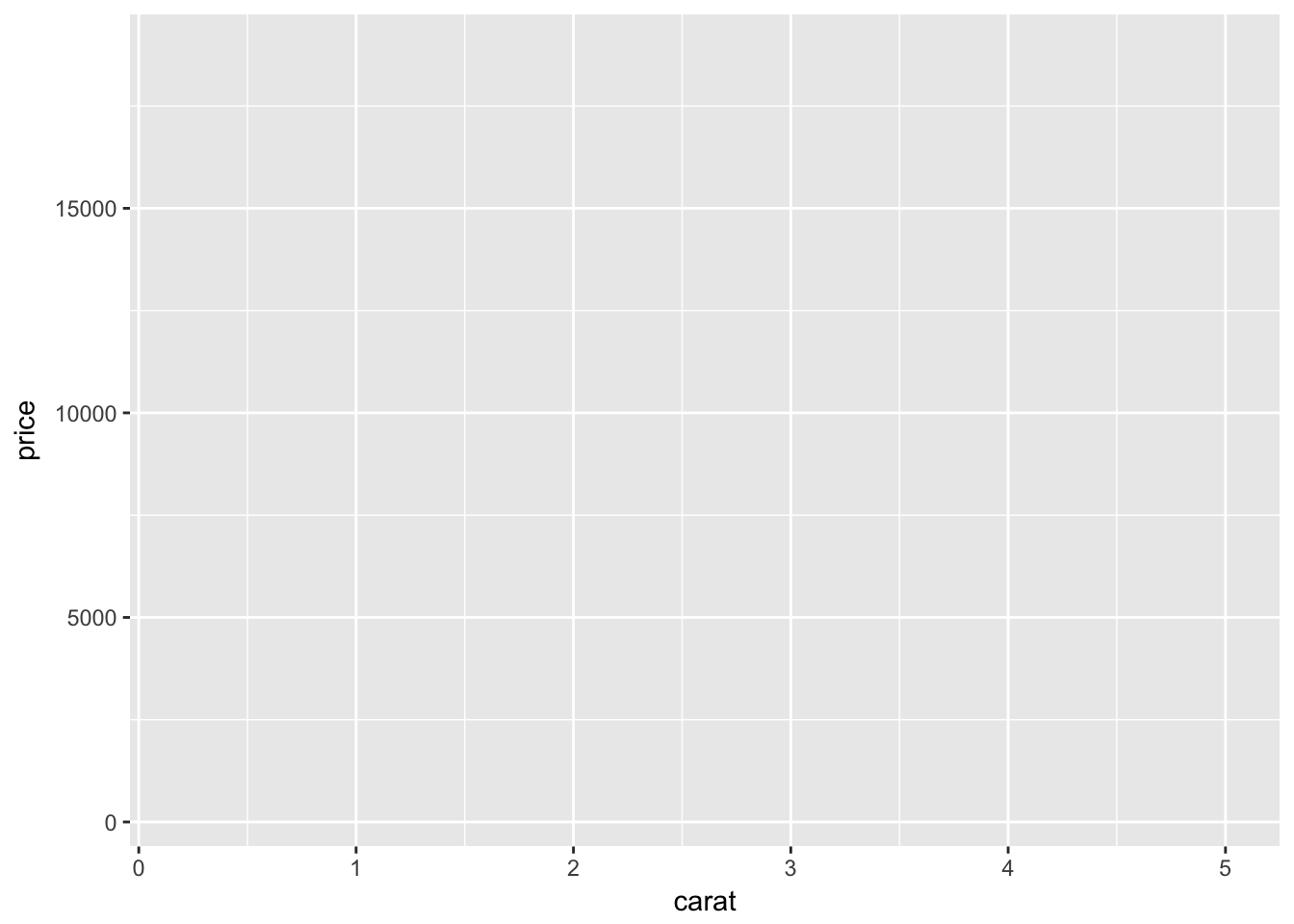## Geometric object: geom_

ggplot(data = diamonds,
aes(x = carat, y = price)) +
geom_point()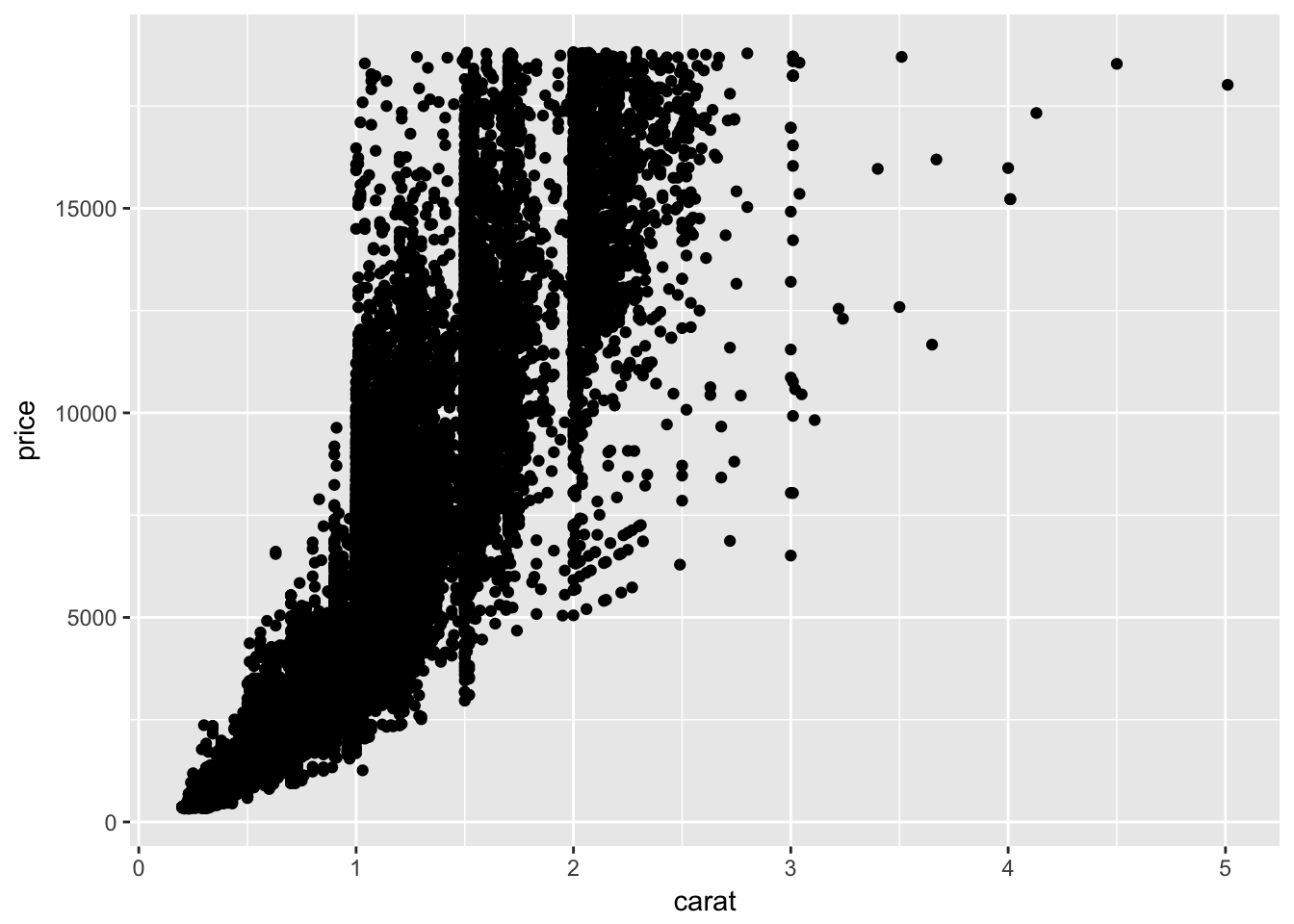## Add more aesthetics…

ggplot(data = diamonds,
aes(x = carat, y = price, color = clarity)) +
geom_point()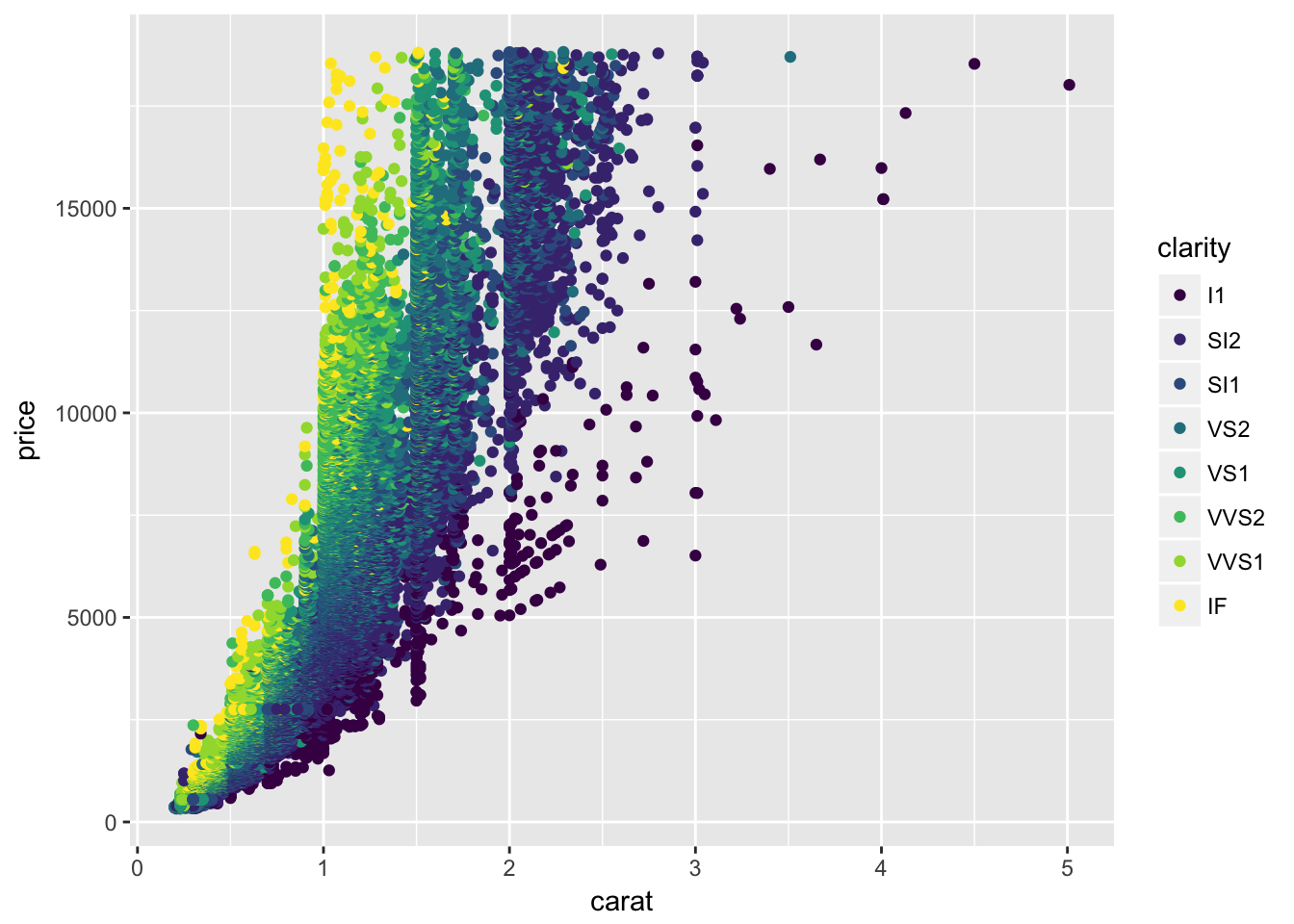## Why didn’t we do this?

ggplot(data = diamonds,
aes(x = carat, y = price), color = clarity) +
geom_point()## We could get this instead:

ggplot(data = diamonds,
aes(x = carat, y = price)) +
geom_point(color = "magenta")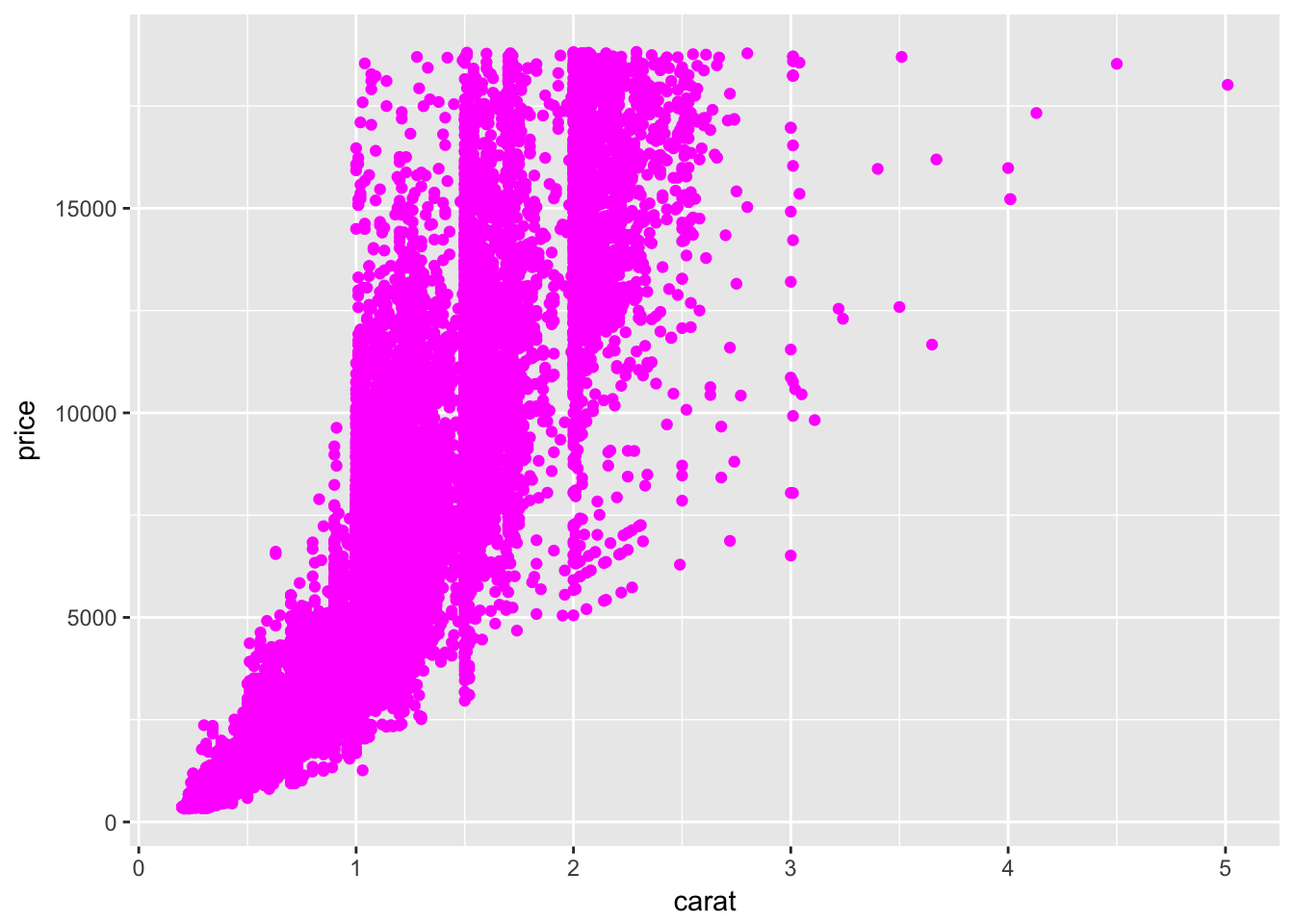## Our original plot

(with a small difference:)

ggplot(data = diamonds,
aes(x = carat, y = price)) +
geom_point(aes(color = clarity))## Add more geoms:

ggplot(data = diamonds,
aes(x = carat, y = price)) +
geom_point(aes(color = clarity)) +
geom_smooth()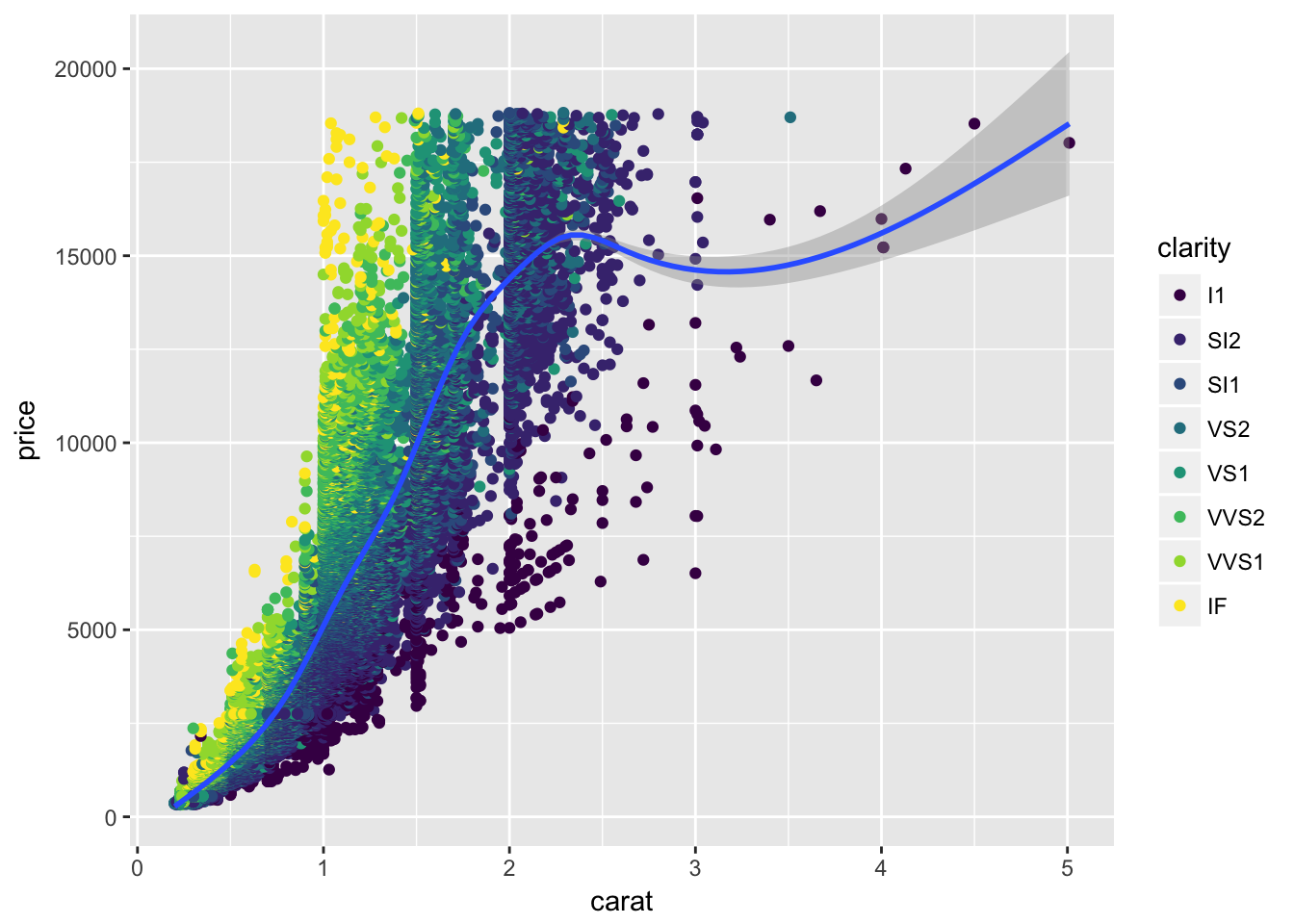## Change geom appearance:

ggplot(data = diamonds,
aes(x = carat, y = price)) +
geom_point(aes(color = clarity)) +
geom_smooth(color = "black", size = 0.8, linetype = 2)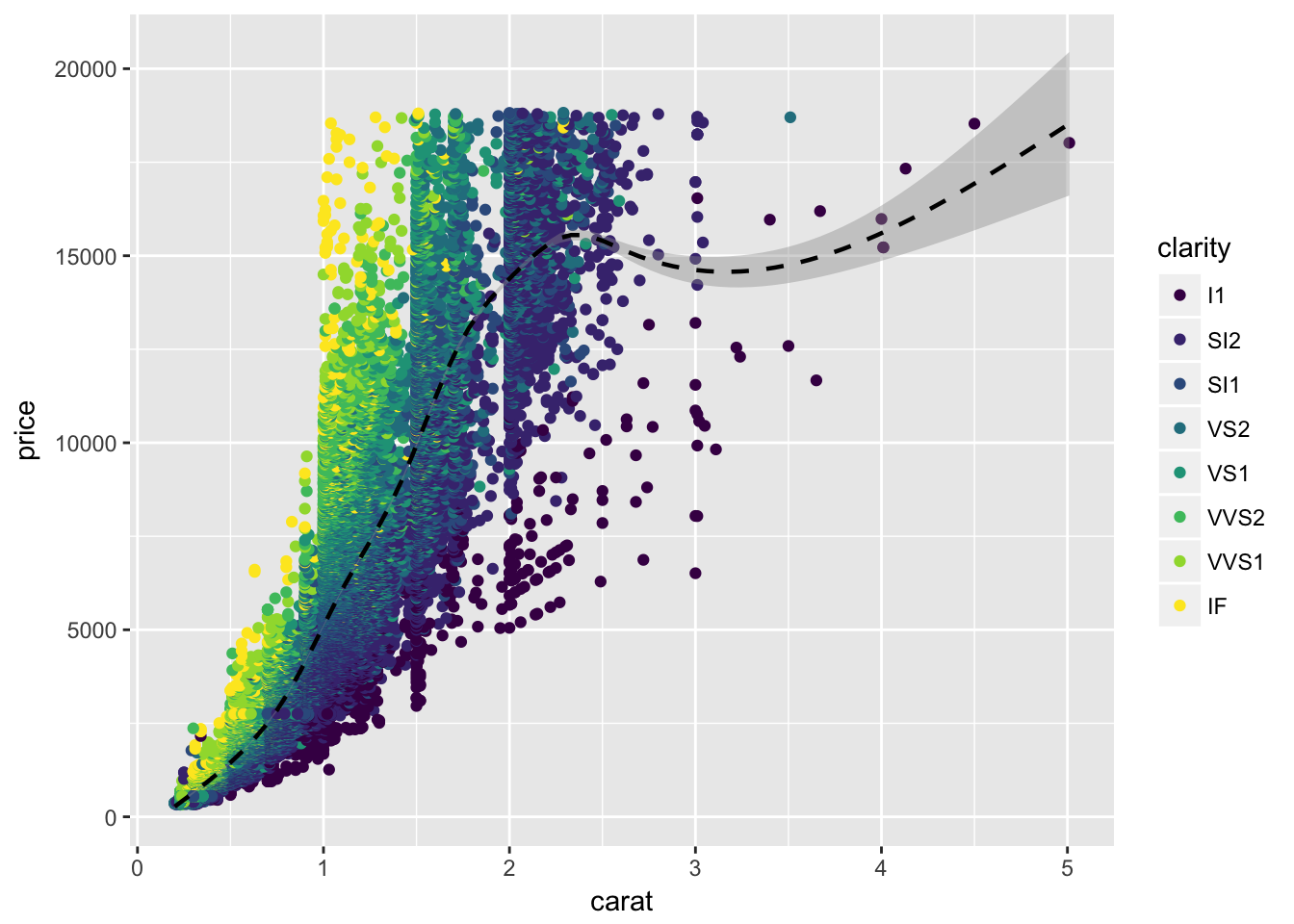## Make it look nicer:

ggplot(data = diamonds,
aes(x = carat, y = price)) +
geom_point(aes(color = clarity)) +
geom_smooth(color = "black", size = 0.8, linetype = 2) +
theme_few()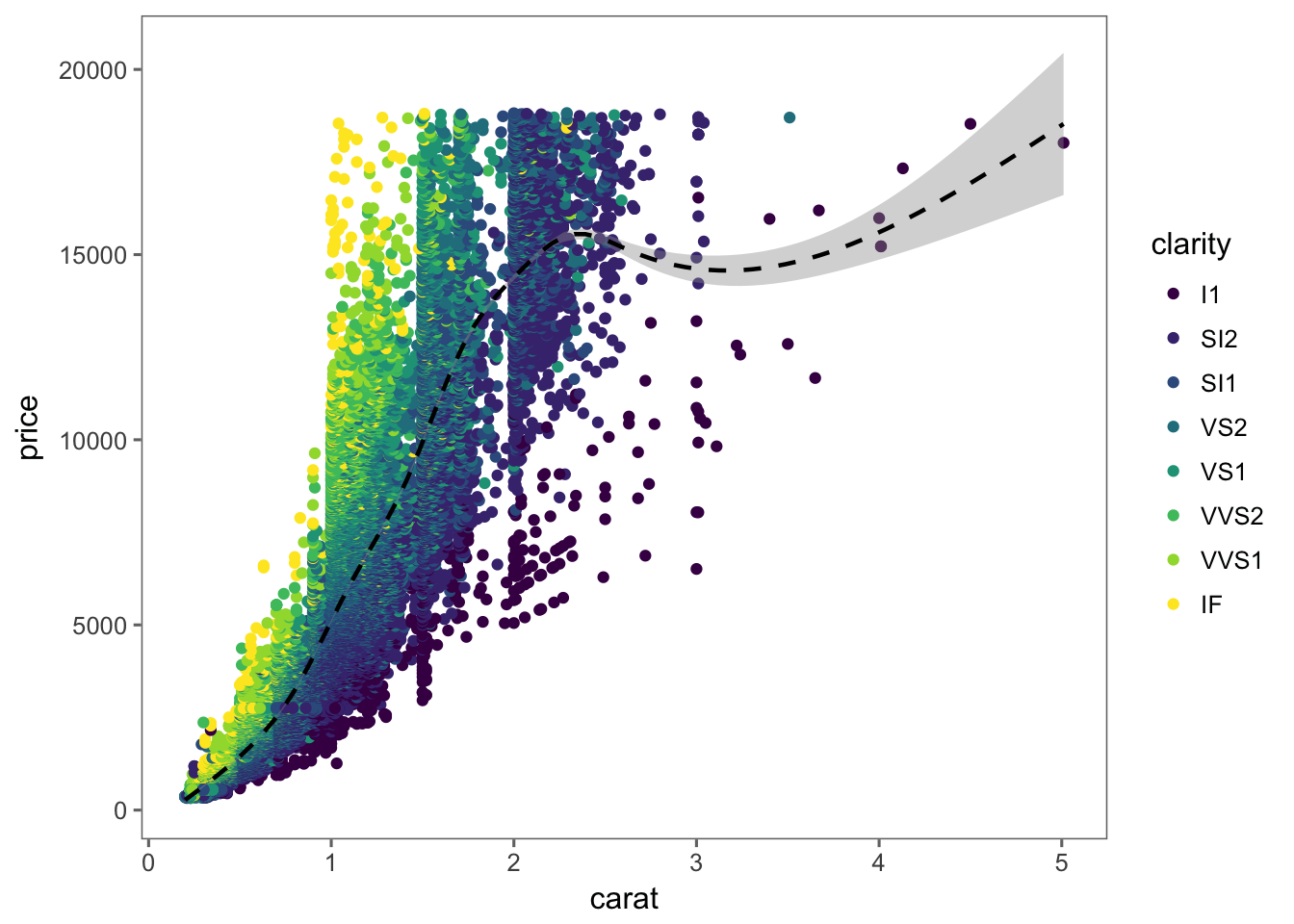## Try facets!

ggplot(data = diamonds,
aes(x = carat, y = price)) +
geom_point(aes(color = clarity)) +
geom_smooth(color = "black", size = 0.8, linetype = 2) +
facet_wrap(~cut)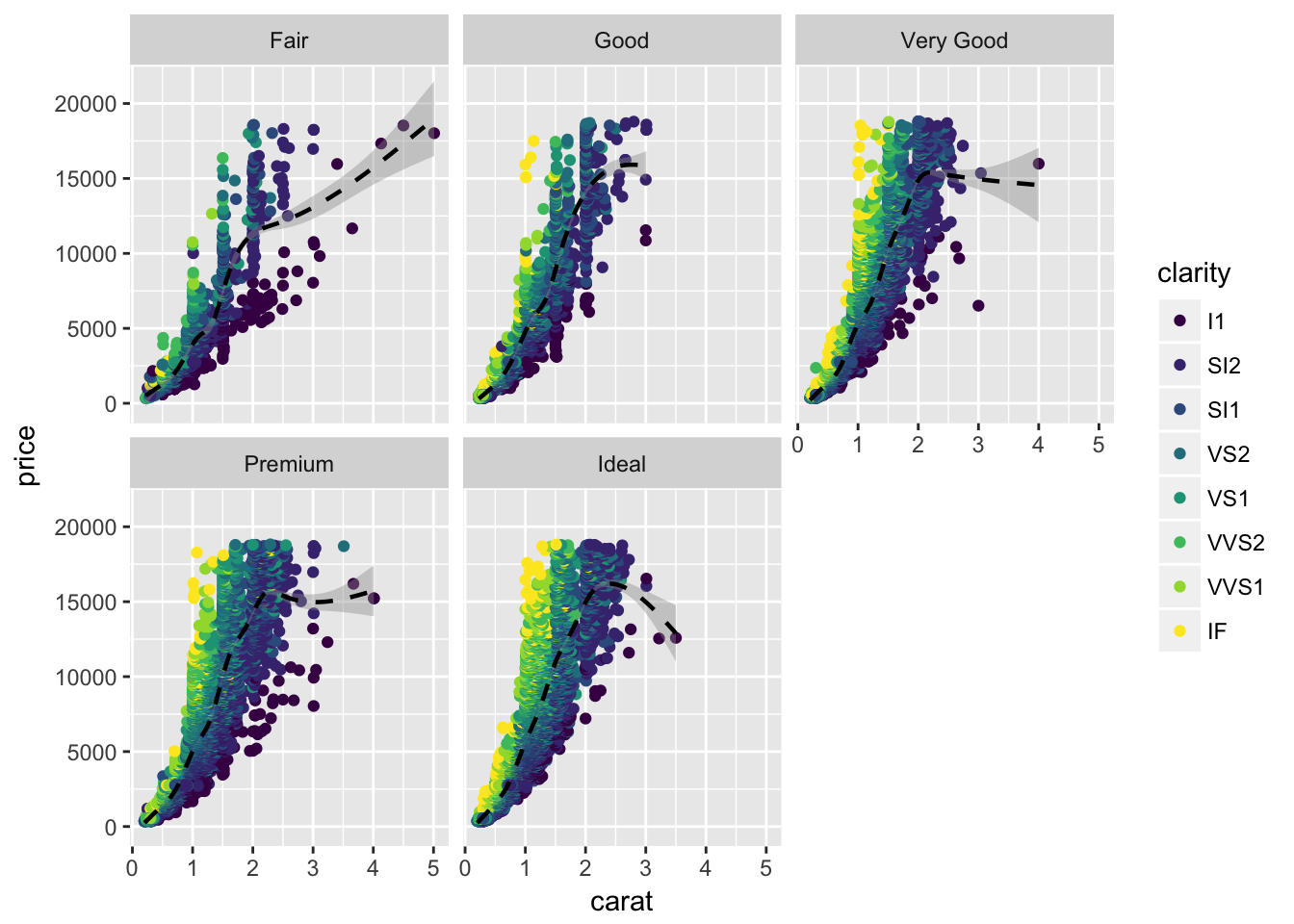## Two-dimensional facets:

ggplot(data = diamonds, aes(x = carat, y = price, color = clarity)) +
geom_point(size = 0.5) +
facet_grid(color~cut)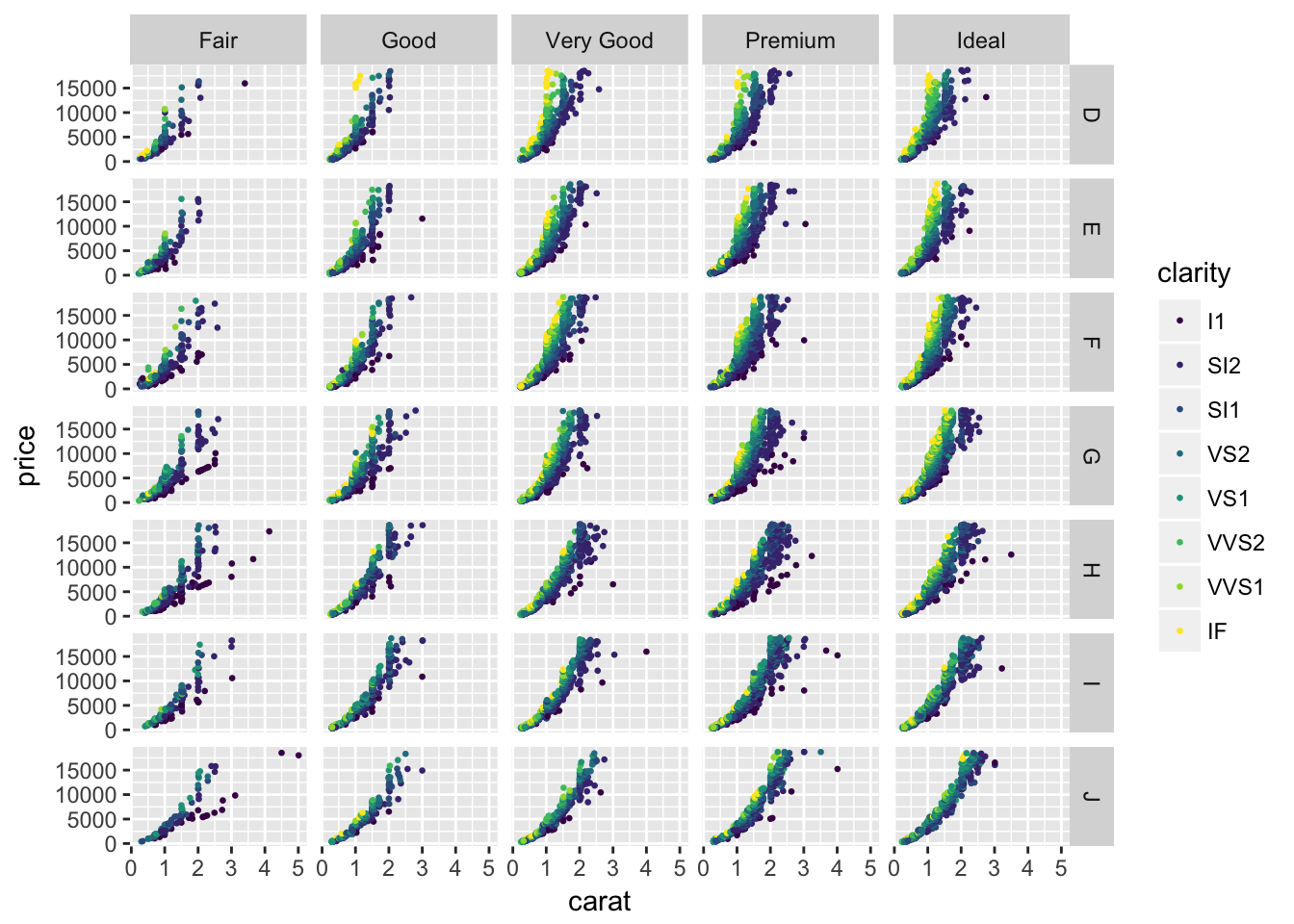## Try out color scales

ColorBrewer is useful and popular:

ggplot(data = diamonds, aes(x = carat, y = price)) +
geom_point(aes(color = clarity)) +
theme_few() +
scale_color_brewer(type = "qual", palette = "Set2")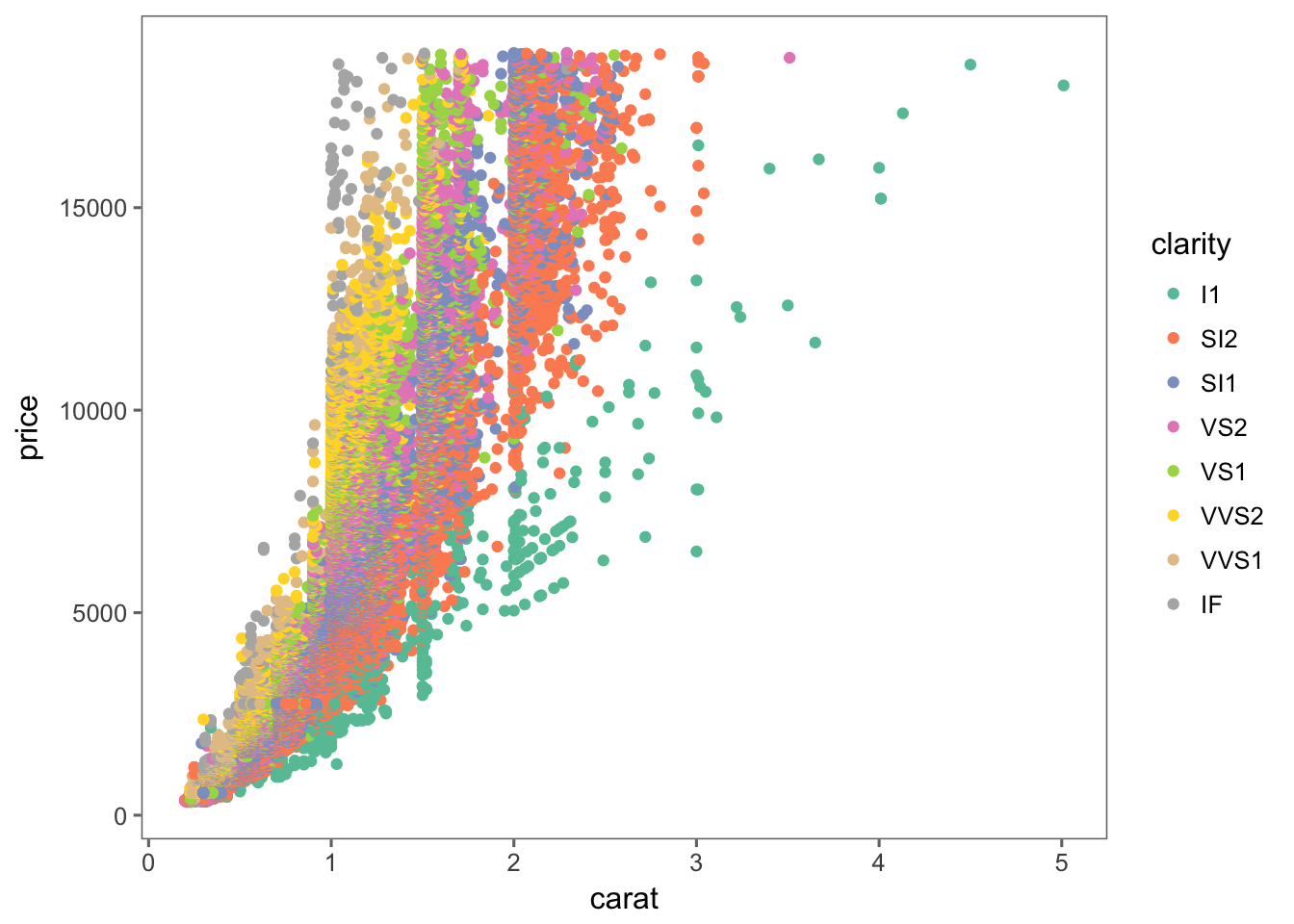## Different plot types?

ggplot(data = diamonds,
aes(x = clarity, y = price)) +
geom_violin()## Try an extension:

ggplot(data = diamonds,
aes(x = price, y = cut)) +
geom_density_ridges()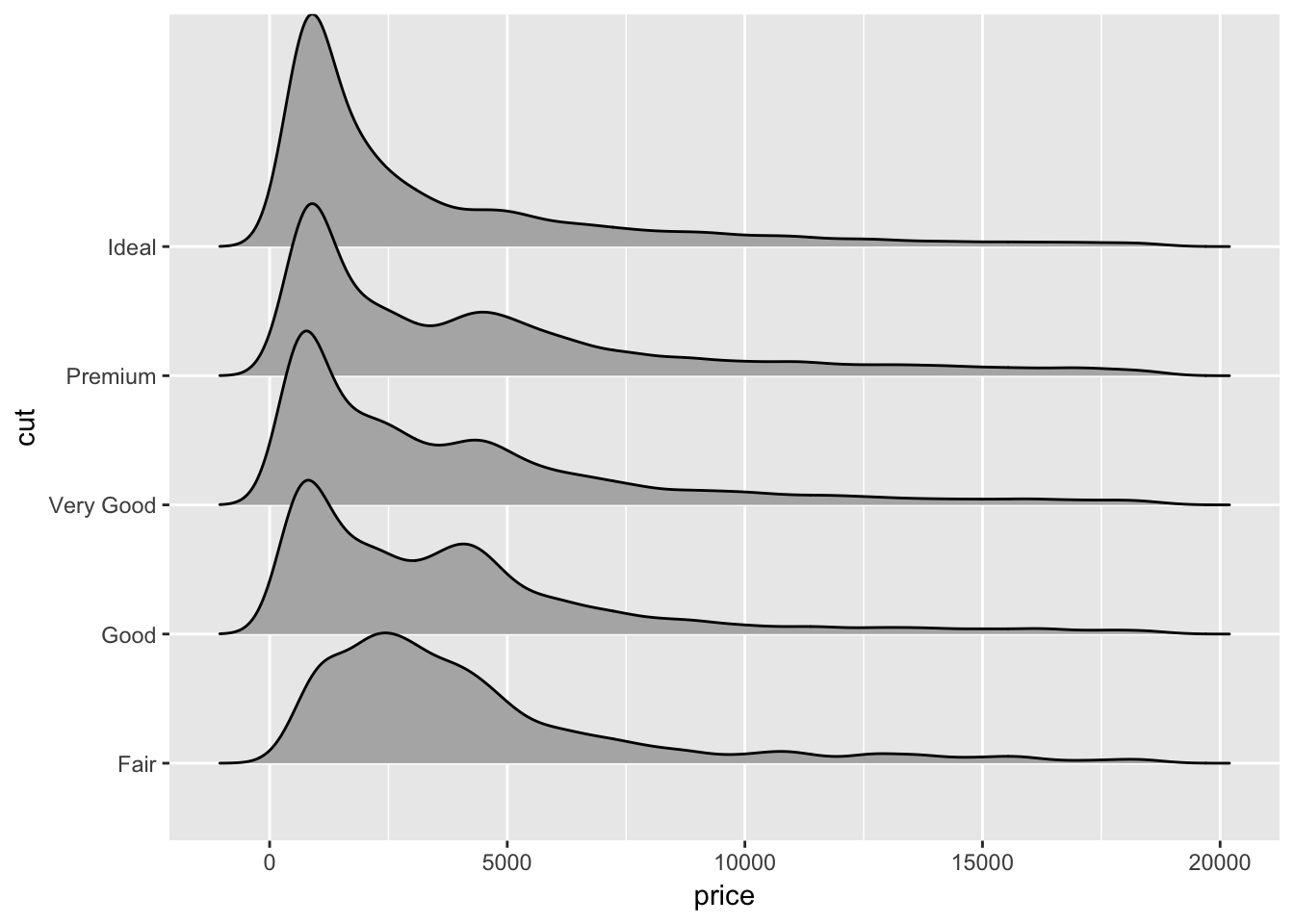## Choose colors: scale_color_…

ggplot(data = diamonds,
aes(x = price, y = cut, color = cut, fill = cut)) +
geom_density_ridges(alpha = 0.8, scale = 5) +
scale_fill_viridis(option = "A", discrete = TRUE) +
scale_color_viridis(option = "A", discrete = TRUE) +
theme_few()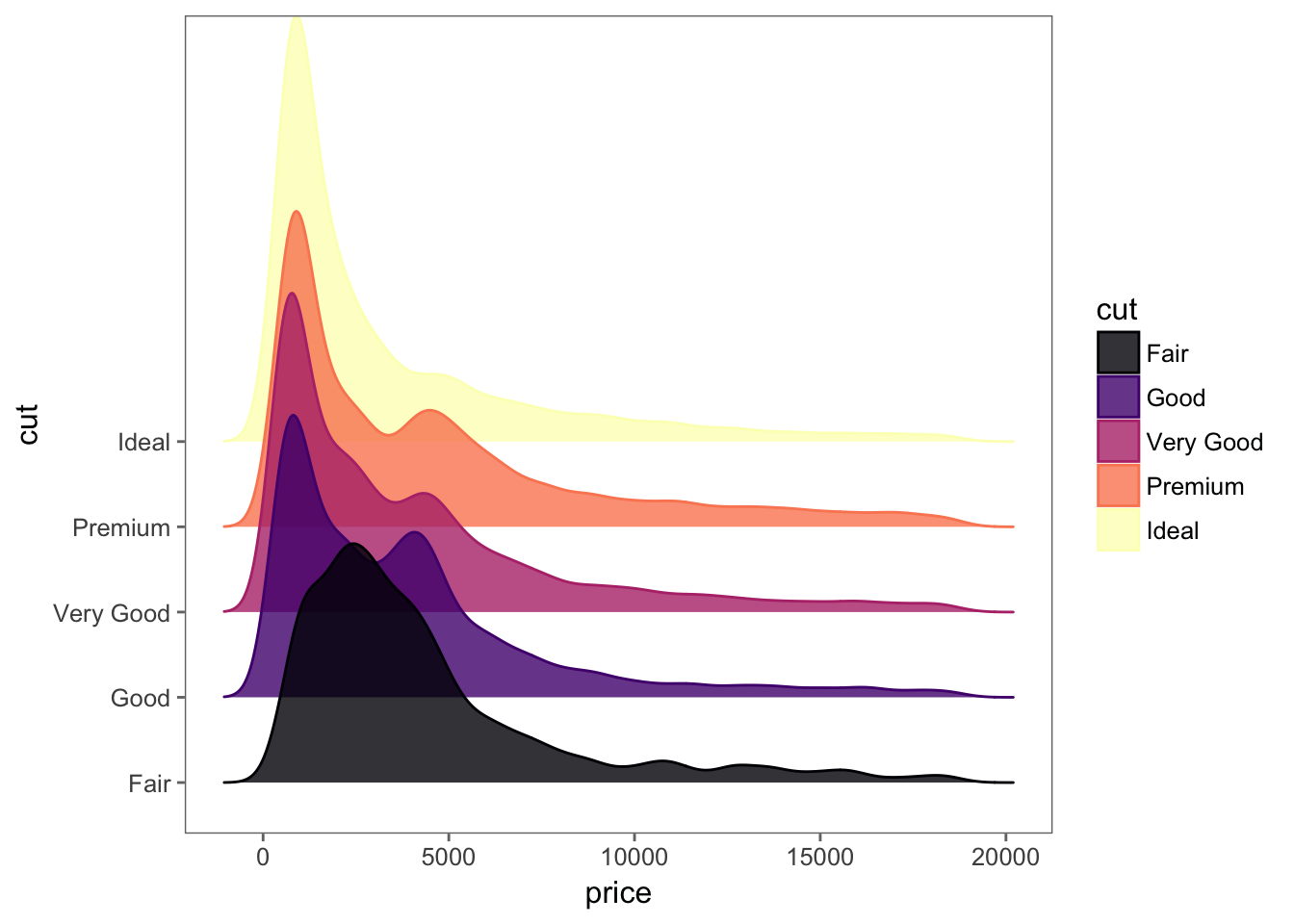## Another color scale:

ggplot(data = diamonds,
aes(x = price, y = cut, fill = cut)) +
geom_density_ridges(alpha = 1, scale = 5) +
scale_fill_manual(values = wes_palette("Darjeeling")) +
theme_few()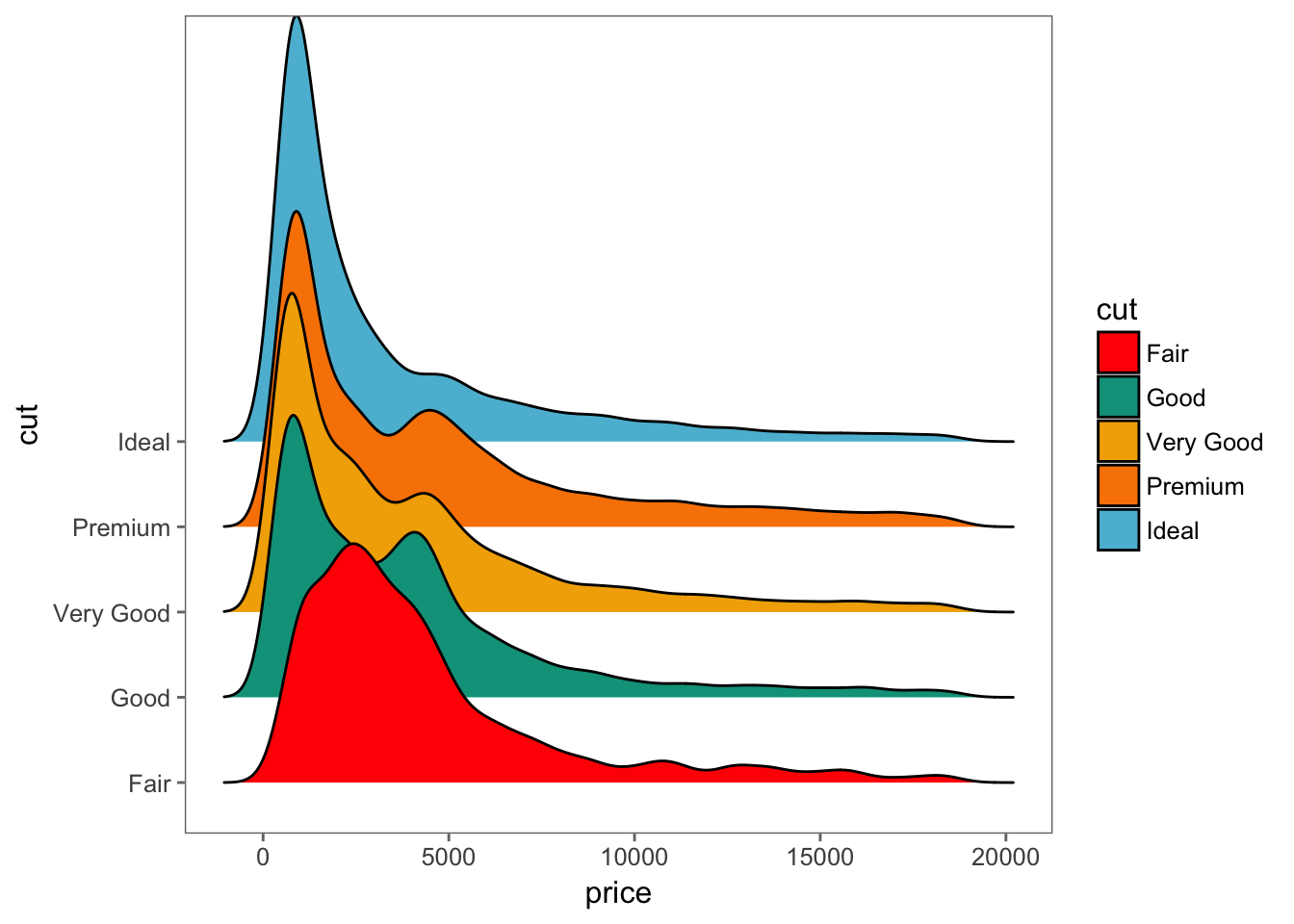## Sometimes we need to transform data.

kable(head(iris))
Sepal.Length Sepal.Width Petal.Length Petal.Width Species
5.1 3.5 1.4 0.2 setosa
4.9 3.0 1.4 0.2 setosa
4.7 3.2 1.3 0.2 setosa
4.6 3.1 1.5 0.2 setosa
5.0 3.6 1.4 0.2 setosa
5.4 3.9 1.7 0.4 setosa

## Wide or long?

iris_long <- melt(iris, id.vars = ("Species"))
kable(head(iris_long))
Species variable value
setosa Sepal.Length 5.1
setosa Sepal.Length 4.9
setosa Sepal.Length 4.7
setosa Sepal.Length 4.6
setosa Sepal.Length 5.0
setosa Sepal.Length 5.4

## Plot the data:

ggplot(iris_long, aes(x = Species, y = value, fill = variable)) +
geom_bar(stat = 'identity', width = 1) +
theme_bw()## Change coordinates

ggplot(iris_long,
aes(x = Species, y = value, color = variable, fill = variable)) +
geom_bar(stat = 'identity', width = 1) +
coord_polar(theta = 'x') +
theme_bw()## A more interesting dataset:

kable(head(gapminder))
country continent year lifeExp pop gdpPercap
Afghanistan Asia 1952 28.801 8425333 779.4453
Afghanistan Asia 1957 30.332 9240934 820.8530
Afghanistan Asia 1962 31.997 10267083 853.1007
Afghanistan Asia 1967 34.020 11537966 836.1971
Afghanistan Asia 1972 36.088 13079460 739.9811
Afghanistan Asia 1977 38.438 14880372 786.1134

## A scatter plot:

ggplot(gapminder, aes(x = gdpPercap, y = lifeExp)) +
geom_point()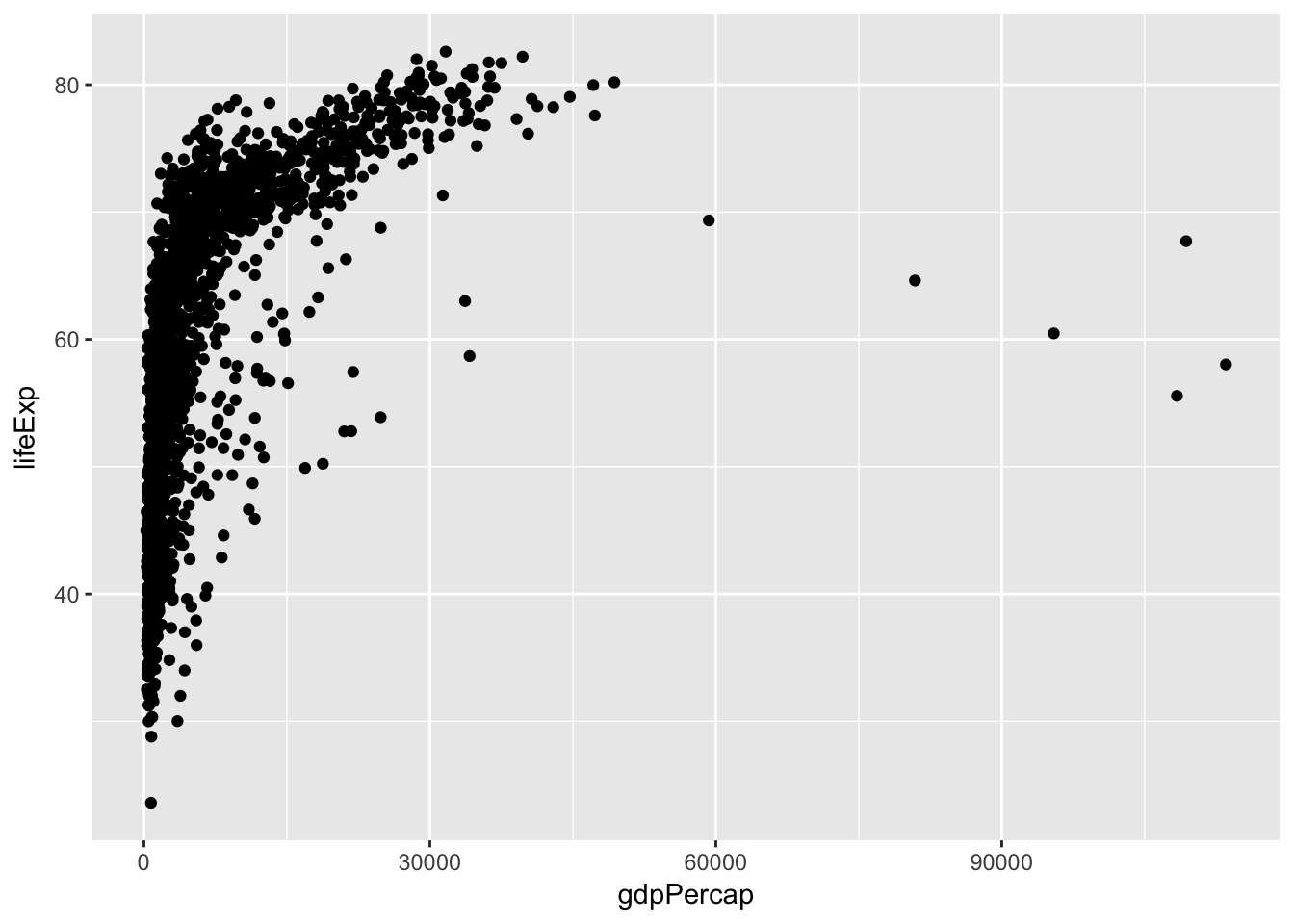Who’s the outlier?

## Who’s the outlier?

gapminder %>% filter(gdpPercap > 60000)
## # A tibble: 5 x 6
##   country continent  year lifeExp    pop gdpPercap
##    <fctr>    <fctr> <int>   <dbl>  <int>     <dbl>
## 1  Kuwait      Asia  1952  55.565 160000 108382.35
## 2  Kuwait      Asia  1957  58.033 212846 113523.13
## 3  Kuwait      Asia  1962  60.470 358266  95458.11
## 4  Kuwait      Asia  1967  64.624 575003  80894.88
## 5  Kuwait      Asia  1972  67.712 841934 109347.87

## Deal with overplotting:

ggplot(gapminder, aes(x = gdpPercap, y = lifeExp)) +
geom_hex()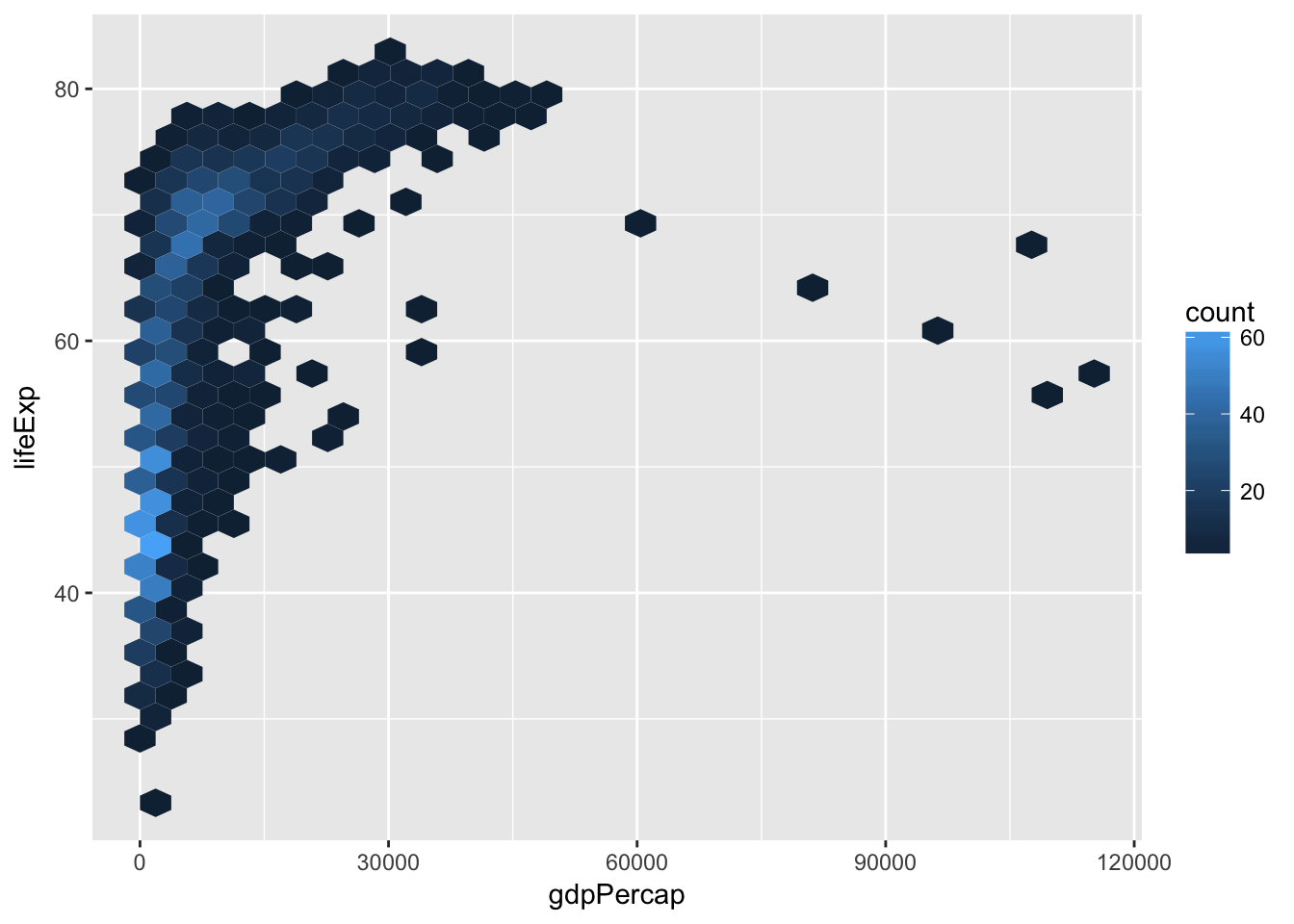## Another way to avoid overplotting:

ggplot(gapminder, aes(x = gdpPercap, y = lifeExp)) +
geom_density2d(aes(color = ..level..), bins = 20) +
scale_color_viridis()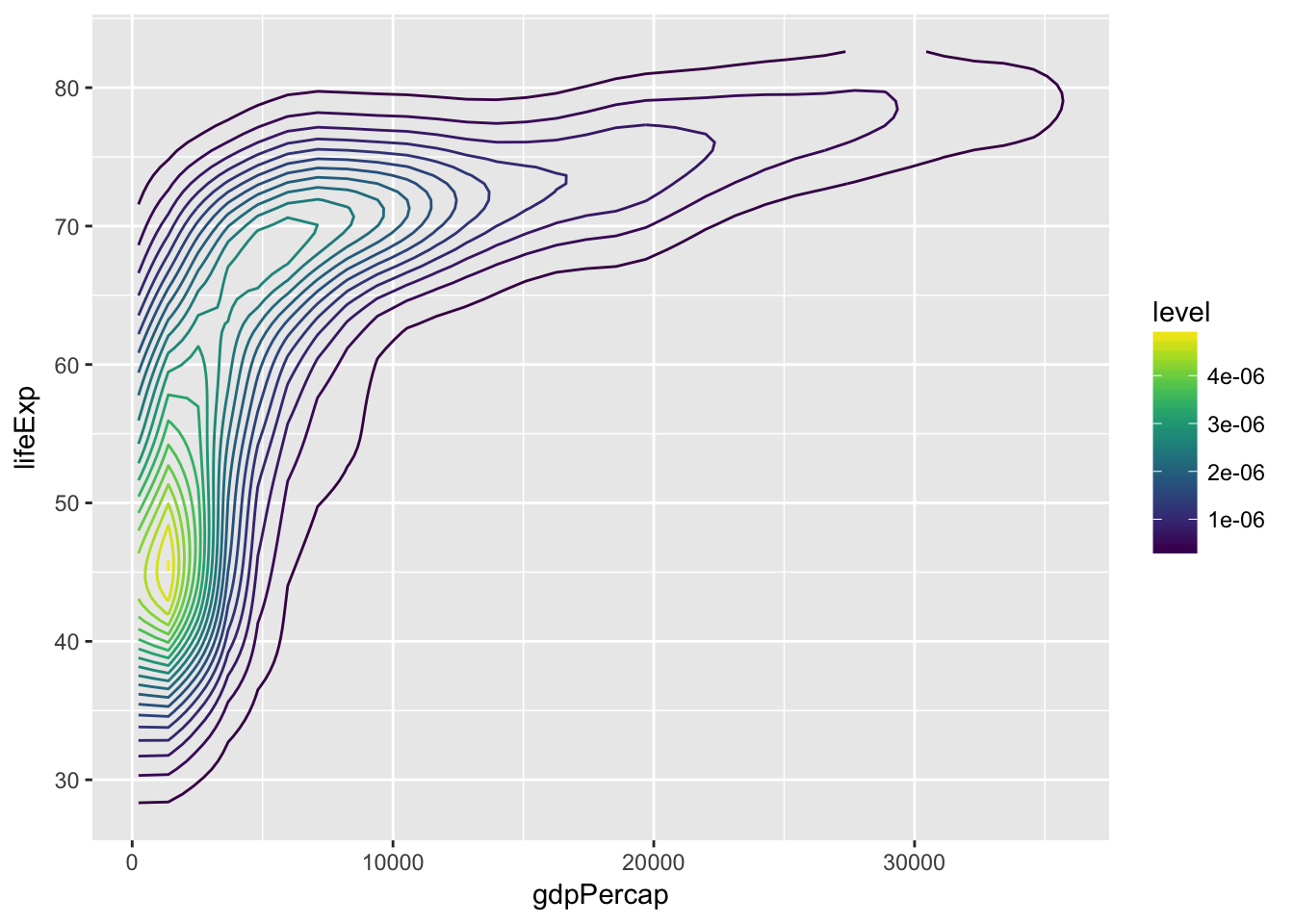## A little more complicated:

p <- ggplot(gapminder, aes(x = gdpPercap, y = lifeExp)) +
geom_point(aes(color = continent, size = pop), alpha = 0.8) +
scale_x_continuous(trans = 'log') +
facet_wrap(~year) +
scale_color_brewer(type = "Qual", palette = "Accent") +
theme_hc(bgc = 'darkunica') +
theme(text = element_text(size = 9))

## A little more complicated:

p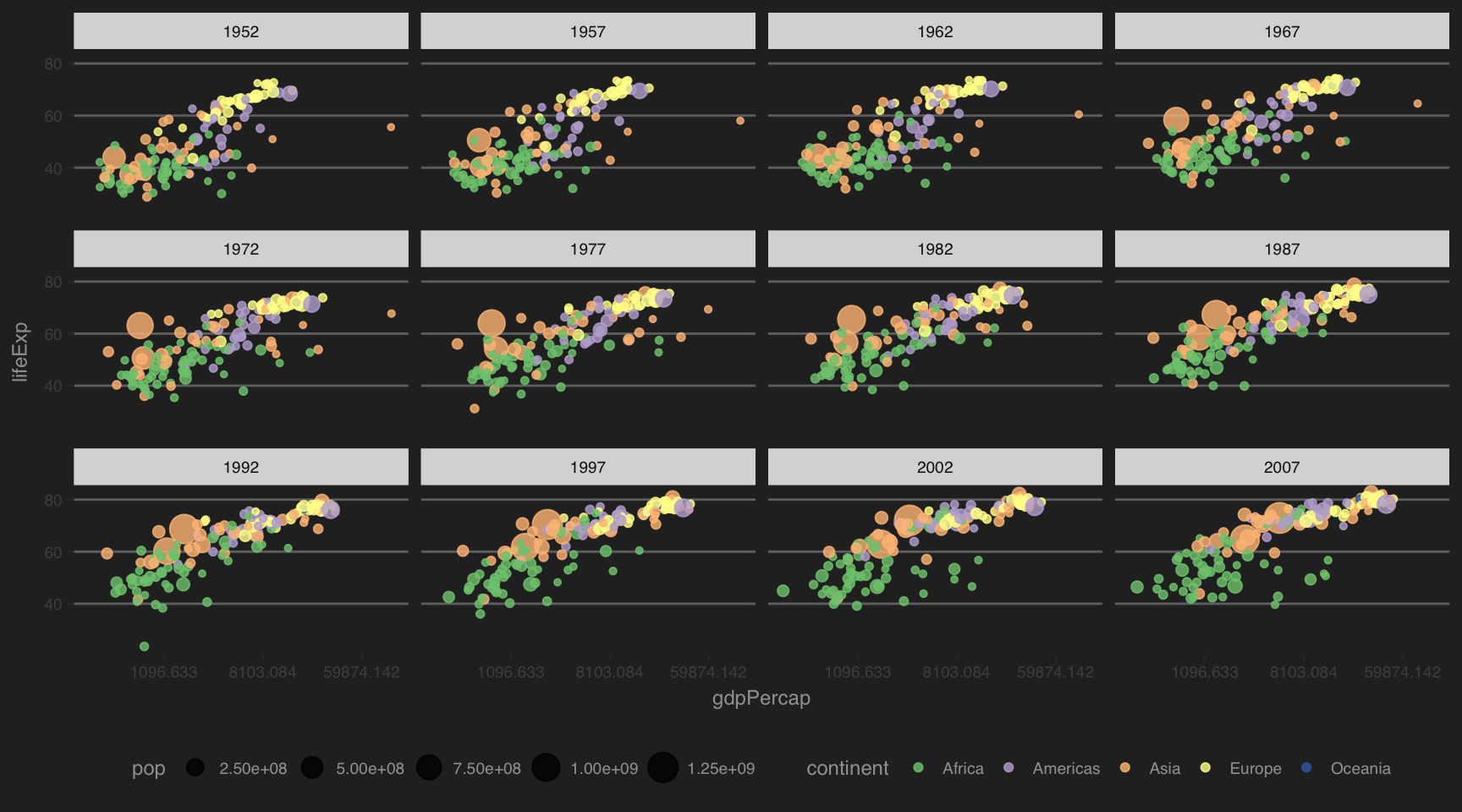## Let’s make a map!

Prepare data:

country_df <- map_data('world') %>%
rename("country" = "region")
country_df$country[country_df$country == "USA"] <- "United States"

#Take the mean across all years for each country:
gapminder_means <- gapminder %>%
group_by(country, continent) %>%
summarise(lifeExp = mean(lifeExp),
pop = mean(pop),
gdpPercap = mean(gdpPercap))

plot_dat <- left_join(gapminder_means, country_df, by = "country")

## Make the map:

ggplot(plot_dat) +
geom_polygon(aes(x = long, y = lat, fill = lifeExp, group = group)) +
scale_fill_viridis(option = "A") +
coord_quickmap() +
theme_few()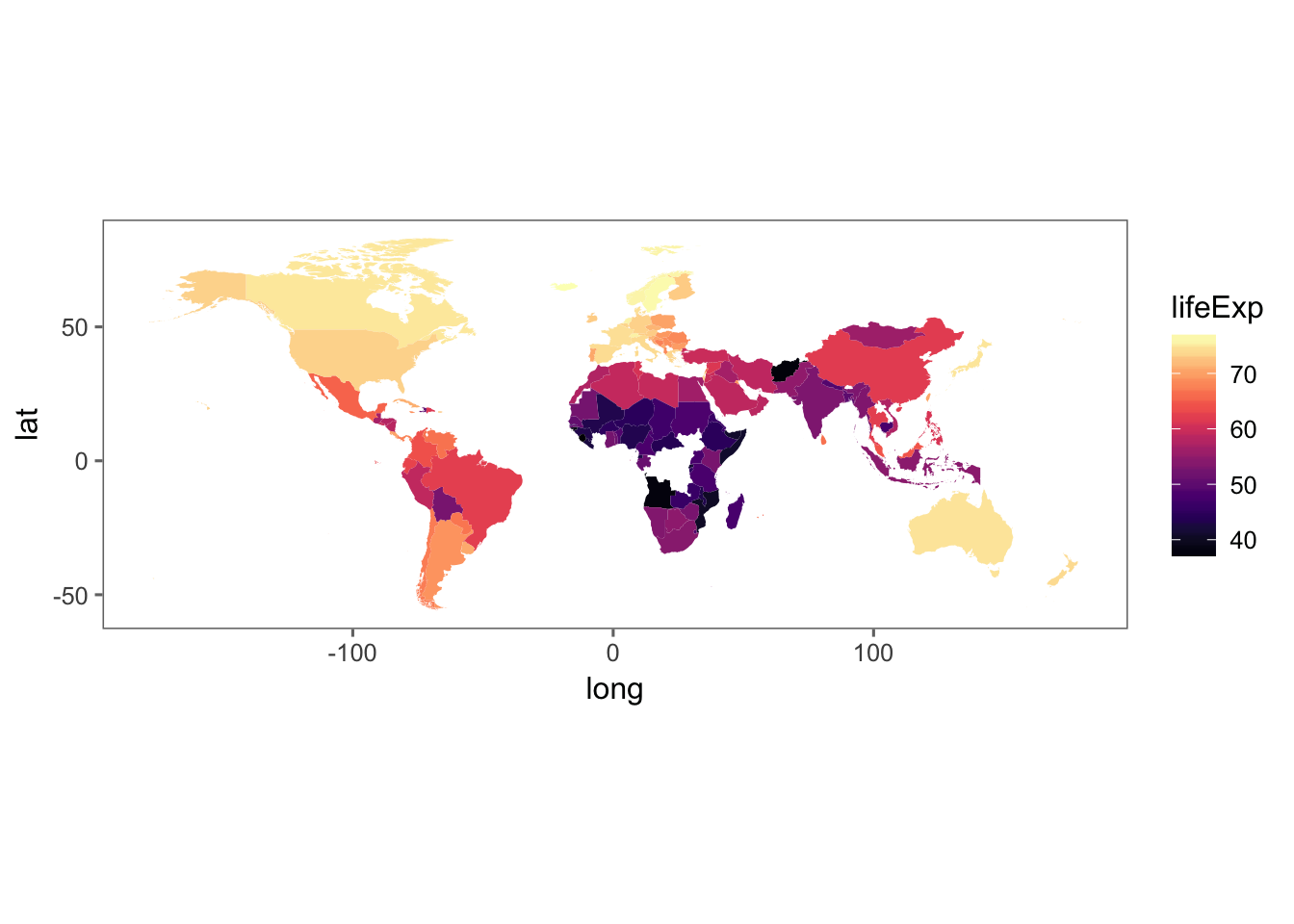## More plot ideas?

• What questions could we ask with this data?

• How could we visually answer those questions?